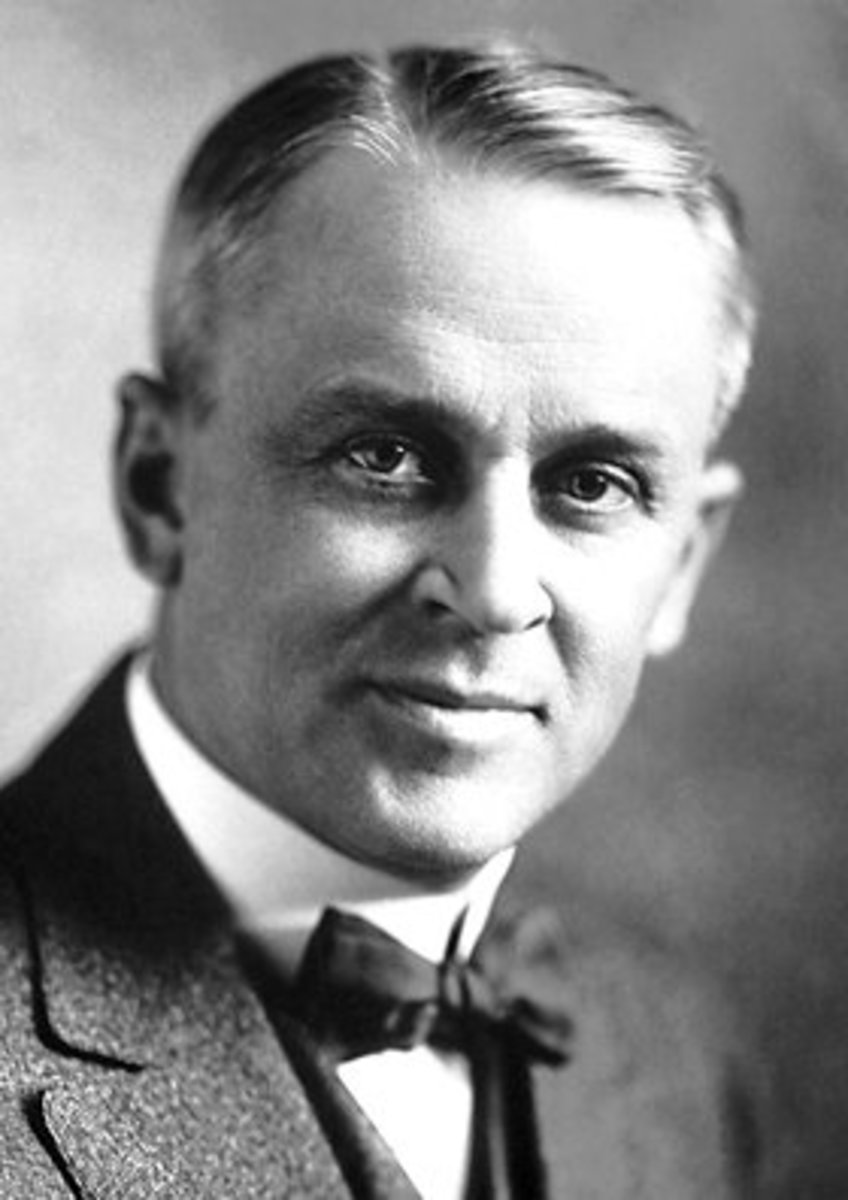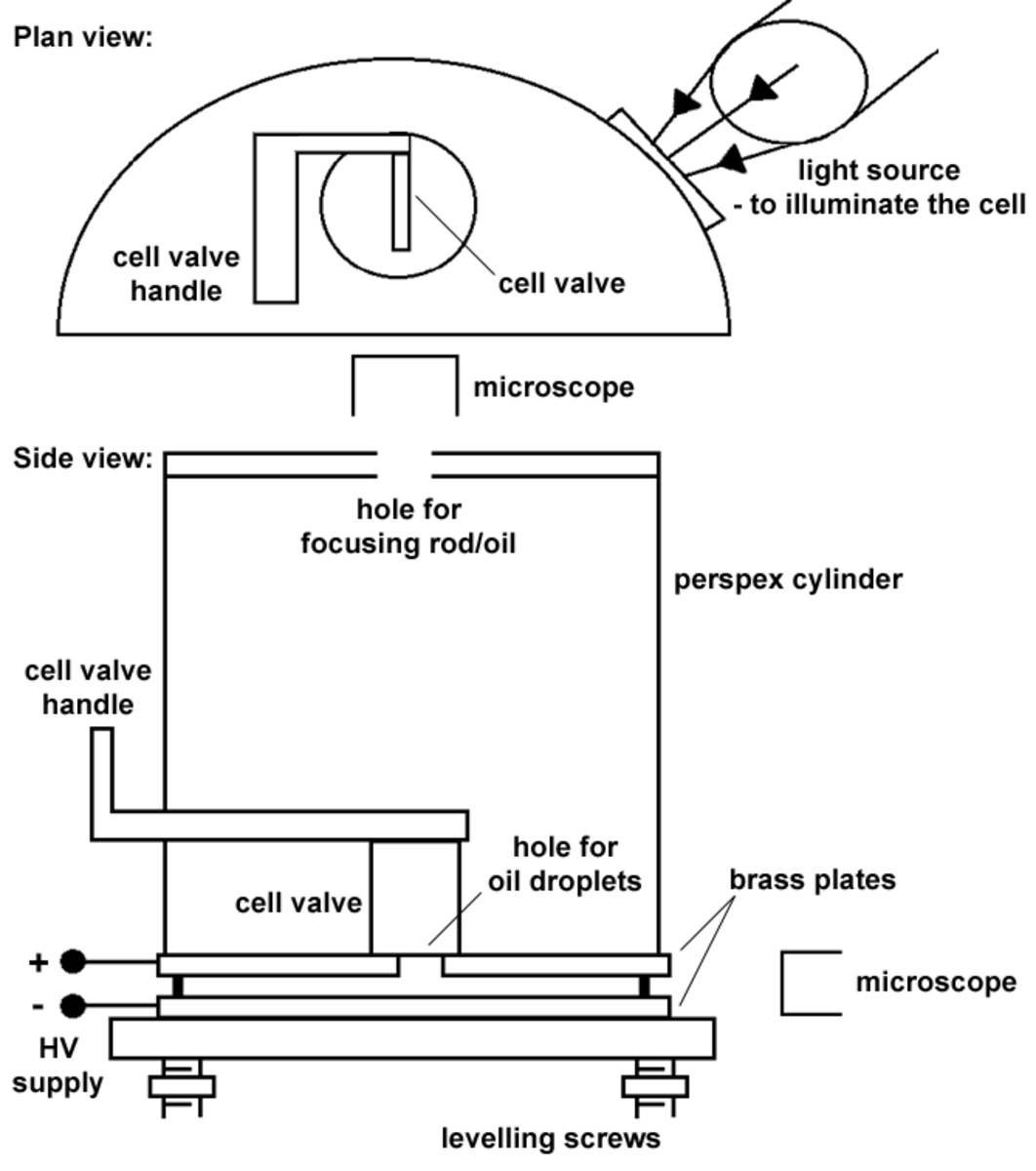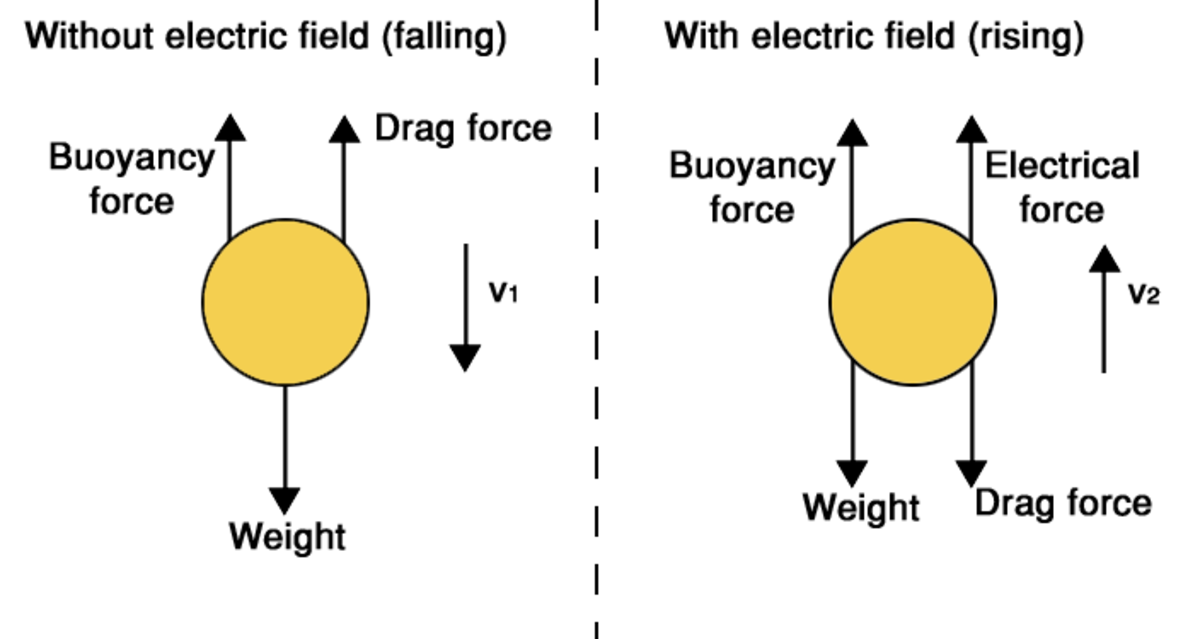# Millikan's Oil Drop Experiment: How to Determine the Charge of an Electron

Sam Brind holds a master's in physics with theoretical physics (MPhys) from the University of Manchester.Robert Millikan, the 1923 Nobel prize winning physicist who determined the electron's charge.Nobelprize.org

## The Discovery of the Electron's Charge

In 1897, J. J. Thomson demonstrated that cathode rays, a new phenomenon, were made up of small, negatively charged particles, which were soon named electrons. The electron was the first subatomic particle ever discovered. Through his cathode ray experiments, Thomson also determined the electrical charge-to-mass ratio for the electron.

Millikan's oil-drop experiment was performed by Robert Millikan and Harvey Fletcher in 1909. It determined a precise value for the electric charge of the electron, e. The electron's charge is the fundamental unit of electric charge because all electric charges are made up of groups (or the absence of groups) of electrons. This discretisation of charge is also elegantly demonstrated by Millikan's experiment.

The unit of electric charge is a fundamental physical constant and crucial to calculations within electromagnetism. Hence, an accurate determination of its value was a big achievement, recognised by the 1923 Nobel prize for physics.

## Millikan's Apparatus

Millikan's experiment is based around observing charged oil droplets in free fall and the presence of an electric field. A fine mist of oil is sprayed across the top of a perspex cylinder with a small 'chimney' that leads down to the cell (if the cell valve is open). The act of spraying will charge some of the released oil droplets through friction with the nozzle of the sprayer. The cell is the area enclosed between two metal plates that are connected to a power supply. Hence an electric field can be generated within the cell, and its strength can be varied by adjusting the power supply. A light is used to illuminate the cell, and the experimenter can observe the cell by looking through a microscope.The apparatus used for Millikan's experiment (shown from two perspectives).

## Terminal Velocity

As an object falls through a fluid, such as air or water, the force of gravity will accelerate the object and speed it up. As a consequence of this increasing speed, the drag force acting on the object that resists the falling also increases. Eventually, these forces will balance (along with a buoyancy force), and therefore the object no longer accelerates. At this point, the object is falling at a constant speed, which is called the terminal velocity. The terminal velocity is the maximum speed the object will obtain while free falling through the fluid.

## The Theory

Millikan's experiment revolves around the motion of individual charged oil droplets within the cell. To understand this motion, the forces acting on an individual oil droplet need to be considered. As the droplets are very small, the droplets are reasonably assumed to be spherical in shape. The diagram below shows the forces and their directions that act on a droplet in two scenarios: when the droplet free falls and when an electric field causes the droplet to rise.The different forces acting on a oil drop falling through air (left) and rising through air due to an applied electric field (right).

The most obvious force is the gravitational pull of the Earth on the droplet, also known as the weight of the droplet. Weight is given by the droplet volume multiplied by the density of the oil (ρoil) multiplied by the gravitational acceleration (g). Earth's gravitational acceleration is known to be 9.81 m/s2, and the density of the oil is usually also known (or could be determined in another experiment). However, the radius of the droplet (r) is unknown and extremely hard to measure.

As the droplet is immersed in the air (a fluid), it will experience an upward buoyancy force. Archimedes' principle states that this buoyancy force is equal to the weight of fluid displaced by the submerged object. Therefore, the buoyancy force acting on the droplet is an identical expression to the weight except that the density of air is used (ρair). The density of air is a known value.

The droplet also experiences a drag force that opposes its motion. This is also called air resistance and occurs as a consequence of friction between the droplet and the surrounding air molecules. Drag is described by Stoke's law, which says that the force depends on the droplet radius, viscosity of air (η) and the velocity of the droplet (v). The viscosity of air is known, and the droplet velocity is unknown but can be measured.

Scroll to Continue

## Read More From Owlcation

When the droplet reaches its terminal velocity for falling (v1), the weight is equal to the buoyancy force plus the drag force. Substituting the previous equations for the forces and then rearranging gives an expression for the droplet radius. This allows the radius to be calculated if v1 is measured.

When a voltage is applied to the brass plates, an electric field is generated within the cell. The strength of this electric field (E) is simply the voltage (V) divided by the distance separating the two plates (d).

If a droplet is charged, it will now experience an electrical force in addition to the three previously discussed forces. Negatively charged droplets will experience an upwards force. This electrical force is proportional to both the electric field strength and the droplet's electrical charge (q).

If the electric field is strong enough, the negatively charged droplets will start to rise from a high enough voltage. When the droplet reaches its terminal velocity for rising (v2), the sum of the weight and drag is equal to the sum of the electrical force and the buoyancy force. Equating the formulae for these forces, substituting in the previously obtained radius (from the fall of the same droplet), and rearranging gives an equation for the droplet's electrical charge. This means that the charge of a droplet can be determined through measurement of the falling and rising terminal velocities, as the rest of the equation's terms are known constants.

## Experimental Method

Firstly, calibration is performed, such as focusing the microscope and ensuring the cell is level. The cell valve is opened, oil is sprayed across the top of the cell, and the valve is then closed. Multiple droplets of oil will now be falling through the cell. The power supply is then turned on (to a sufficiently high voltage). This causes negatively charged droplets to rise but also makes positively charged droplets fall quicker, clearing them from the cell. After a very short time, this only leaves negatively charged droplets remaining in the cell.

The power supply is then turned off, and the drops begin to fall. A droplet is selected by the observer, who is watching through the microscope. Within the cell, a set distance has been marked, and the time for the selected droplet to fall through this distance is measured. These two values are used to calculate the falling terminal velocity. The power supply is then turned back on, and the droplet begins to rise. The time to rise through the selected distance is measured and allows the rising terminal velocity to be calculated. This process could be repeated multiple times and allow average fall and rise times, and hence velocities, to be calculated. With the two terminal velocities obtained, the droplet's charge is calculated from the previous formula.

## Results

This method for calculating a droplet's charge was repeated for a large number of observed droplets. The charges were found to all be integer multiples (n) of a single number, a fundamental electric charge (e). Therefore, the experiment confirmed that the charge is quantised.

A value for e was calculated for each droplet by dividing the calculated droplet charge by an assigned value for n. These values were then averaged to give a final measurement of e.

Millikan obtained a value of -1.5924 x 10-19 C, which is an excellent first measurement considering that the currently accepted measurement is -1.6022 x 10-19 C.

## Questions & Answers

Question: Why do we use oil and not water when determining the charge of an electron?

Answer: Millikan needed a liquid to produce droplets that would maintain their mass and spherical shape throughout the course of the experiment. To allow the droplets to be clearly observed, a light source was used. Water was not a suitable choice as water droplets would have begun evaporating under the heat of the light source. Indeed, Millikan chose to use a special type of oil that had a very low vapor pressure and would not evaporate.

Question: How was the value of 'n' calculated for the problem described in this article?

Answer: After performing the experiment, a histogram of electrical charges from the observed droplets is plotted. This histogram should roughly show a pattern of equally spaced clusters of data (demonstrating a quantized charge). Droplets within the lowest value cluster are assigned an 'n' value of one, droplets within the next lowest value cluster are assigned an 'n' value of two and so on.

Question: What is the acceleration of the droplet if the electric force is equal but opposite to that of gravity?

Answer: If the electrical force exactly balances the force of gravity the oil droplet's acceleration will be zero, causing it to float in mid-air. This is actually an alternative to the method of observing the droplet rise in an electric field. However, it is much more difficult to realize these conditions and observe a floating droplet, as it will still be undergoing random motion as a result of collisions with air molecules.

Question: How do the oil droplets acquire either the negative or the positive charge?

Answer: The electrical charge of the oil droplets is a convenient byproduct of how the oil is inserted into the cell. Oil is sprayed into the tube, during this spraying process some of the droplets will obtain a charge through friction with the nozzle (similar to the effect of rubbing a balloon on your head). Alternatively, the droplets could be given a charge by exposing the droplets to ionizing radiation.

© 2017 Sam Brind

Nwizzi chinwendu on January 29, 2020:

The best explanation

Wafula Eric on January 04, 2020:

Thanks for this well-detailed explanation. I really adored it

Ryan on October 21, 2019:

One of the best explanation to the topic.

Detailed and most importantly well structured and presented.

paul chukwualuka on September 25, 2019:

quite elaborate and detailed

samuel mutukiu on July 12, 2019:

its a wonderful explanation .The basis of Millikan's experiment is openly understood.

keith2019 on March 19, 2019:

It is a beautiful introduction about oil drop experiment. From background, theory to data analyses, it is quite clear.

Sam Brind (author) on March 08, 2019:

Like all other scientific experiments, the quantities involved are measured in SI units. For example: masses are measured in kilograms (kg), distances are measured in metres (m), forces are measured in Newtons (N) and electrical charges are measured in Coulombs (C).

ASE DAVID Alabokurogha on March 07, 2019:

good one but I still

need to know the basic units

professor kasirye on September 23, 2018:

this is really wonderful, I just liked it's simplicity